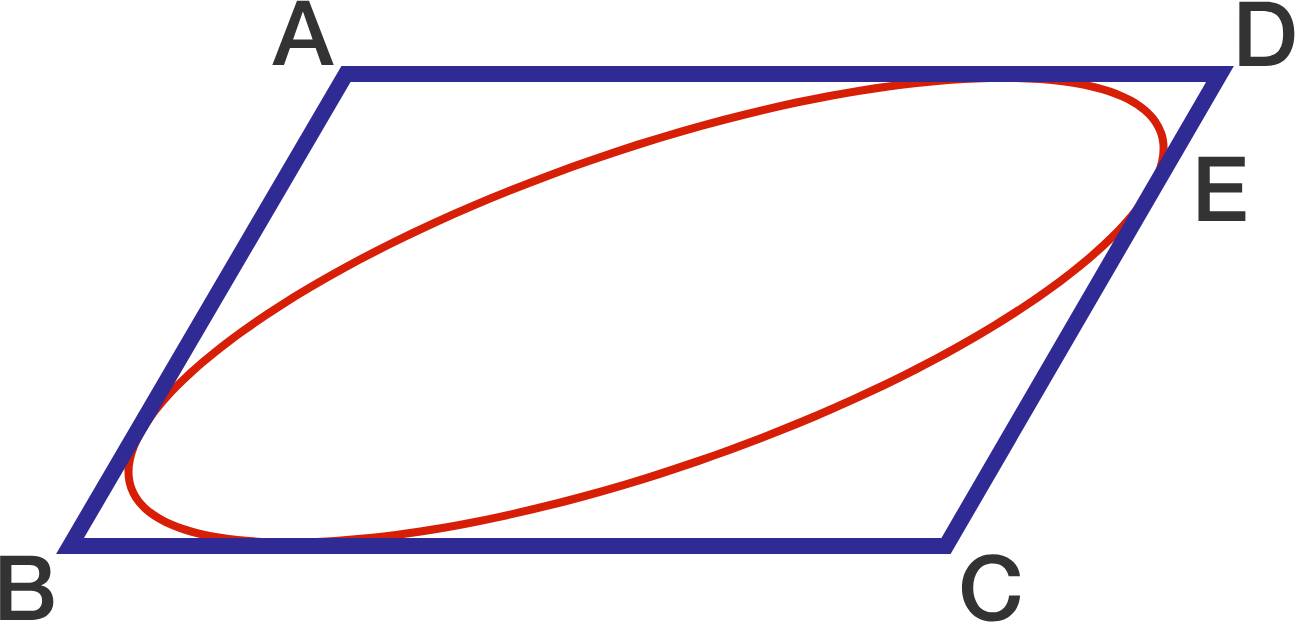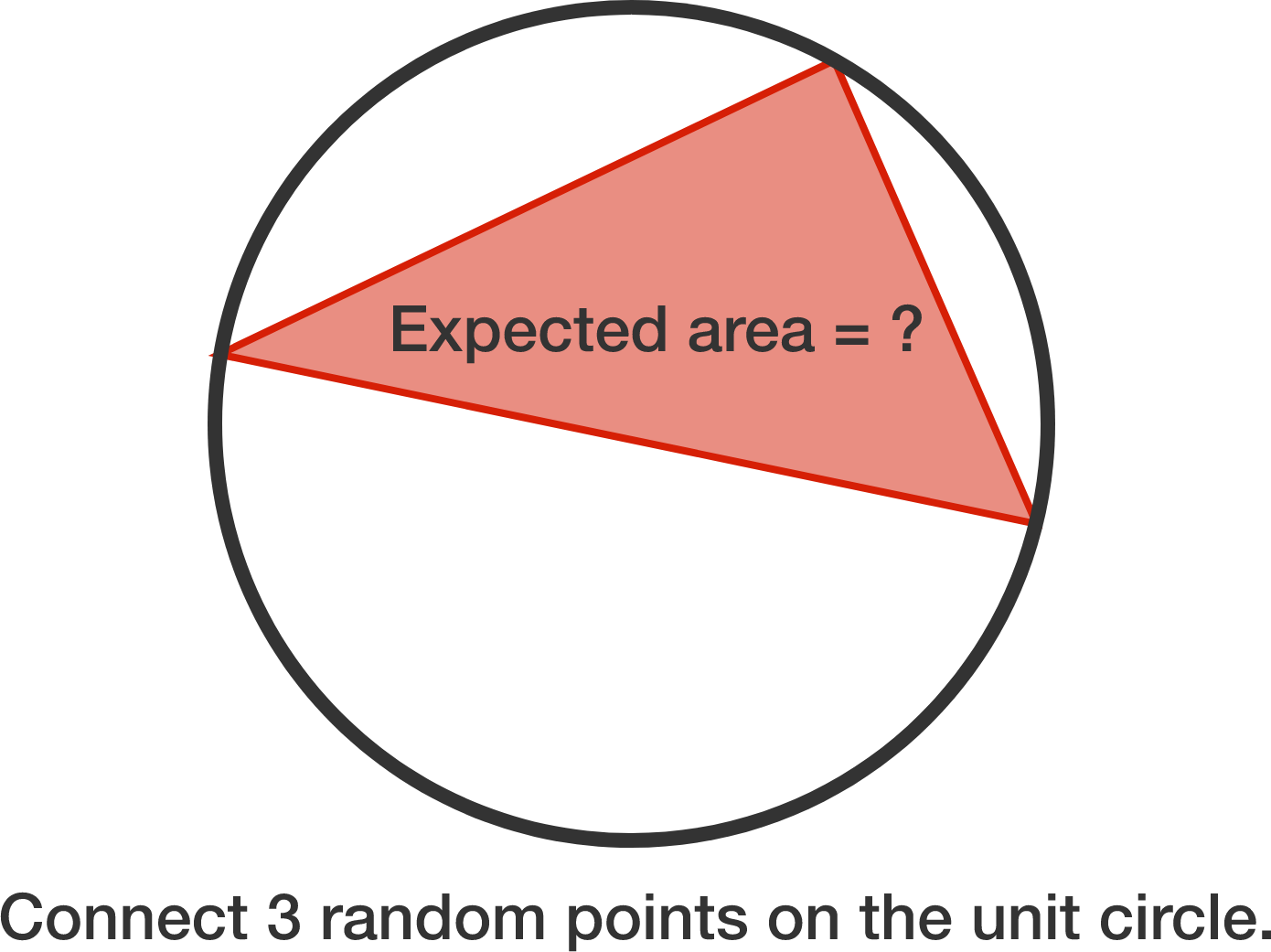# Problems of the Week

Contribute a problem

# 2017-06-26 Advanced

True or False?

The infinite sequence $\big\lfloor 1\times \sqrt{ 2017 } \big\rfloor,\ \big\lfloor 2 \times \sqrt{ 2017 } \big\rfloor,\ \big\lfloor 3 \times \sqrt{ 2017 } \big\rfloor,\ \big\lfloor 4 \times \sqrt{ 2017 } \big\rfloor,\ \ldots$ contains infinitely many perfect squares.


Notation: $\lfloor \cdot \rfloor$ denotes the floor function.

Pi Han has written down a perfect square between 1 to 300 inclusive. Calvin wants to know the integer, and is allowed to ask "yes/no" questions to determine it. Pi Han would immediately answer the question, and then Calvin can use that information to ask his next question. As a point of pride, Calvin would not ask questions to which he already knows the answer. Calvin asks the following questions:

1. Is it less than 100?
2. Is it even?
3. Is it 2-digit?
4. Is the second digit from the left a 6?

At this point, Calvin declares that he knows the answer. However, he turns out to be incorrect because Pi Han gave the wrong answer to each of the 4 questions!

What is the number in the envelope? If you think it cannot be uniquely determined, enter 0 as your answer.


Clarification: Before the questions are asked, Calvin knows that Pi Han wrote down a perfect square between 1 and 300 inclusive.Parallelogram $ABCD$ with $AB = 10, BC =16, \angle ABC = 60^{\circ}$ has an inscribed ellipse such that the tangent point $E$ satisfies $CE = \frac{3}{4} CD$.

Find the length of the semi-major axis of this ellipse.

Three points are chosen at random on the circumference of a circle with radius 1. Find the expected value, $v$, of the area of the triangle formed by the three points.

If $v = \dfrac{a}{b \pi}$, where $a$ and $b$ are coprime positive integers, submit $a+b$.$\frac{r^2}{s}+200=\frac{s^2}{r}+200^2$

In the equation above, $r$ and $s$ are both rational numbers and their difference is an integer.

What is the number of solutions to the equation?

×

Problem Loading...

Note Loading...

Set Loading...